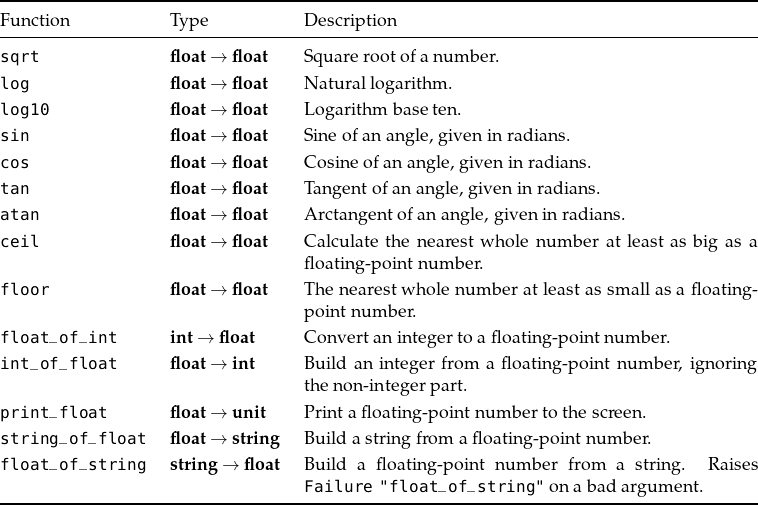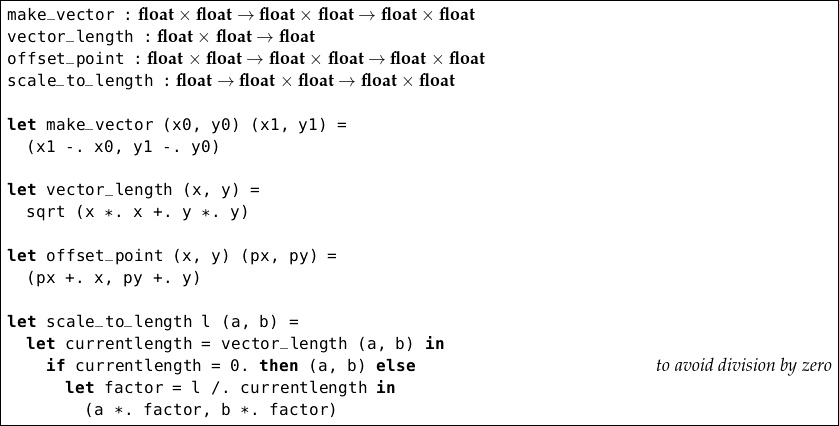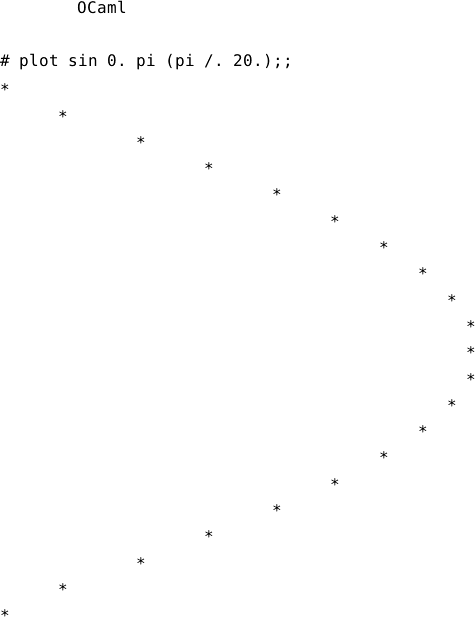Back to Contents

# The Other Numbers

The only numbers we have considered until now have been the integers. For a lot of programming tasks, they are sufficient. And, except for their limited range and the possibility of division by zero, they are easy to understand and use. However, we must now consider the real numbers.

It is clearly not possible to represent all numbers exactly – they might be irrational like π or e and have no finite representation. For most uses, a representation called floating-point is suitable, and this is how OCaml’s real numbers are stored. Not all numbers can be represented exactly, but arithmetic operations are very quick.

Floating-point numbers have type float. We can write a floating-point number by including a decimal point somewhere in it. For example `1.6` or `2.`  or `386.54123`. Negative floating-point numbers are preceded by the `-.` characters just like negative integers are preceded by the `-` character. Similarly, we write `+.` `-.` `*.` `/.` for the standard arithmetic operators on floating-point numbers. Exponentiation is written with the `**` operator.

` OCaml`

`# 1.5;;`
`- : float = 1.5`
`# 6.;;`
`- : float = 6.`
`# -.2.3456;;`
`- : float = -2.3456`
`# 1.0 +. 2.5 *. 3.0;;`
`- : float = 8.5`
`# 1.0 /. 1000.0;;`
`- : float = 0.001`
`# 1. /. 100000.;;`
`- : float = 1e-05`
`# 3000. ** 10.;;`
`- : float = 5.9049e+34`
`# 3.123 -. 3.;;`
`- : float = 0.12300000000000022`

Notice an example of the limits of precision in floating-point operations in the final lines. Note also that very small or very large numbers are written using scientific notation (such as `5.9049e+34` above). We can find out the range of numbers available:

` OCaml`

`# max_float;;`
`- : float = 1.79769313486231571e+308`
`# min_float;;`
`- : float = 2.22507385850720138e-308`

Working with floating-point numbers requires care, and a comprehensive discussion is outside the scope of this book. These challenges exist in any programming language using the floating-point system. For example, evaluating `1. /. 0.` gives the special value `infinity` (there is no `Division_by_zero` exception for floating-point operations). There are other special values such as `neg_infinity` and `nan` (“not a number”). We will leave these complications for now – just be aware that they are lurking and must be confronted when writing robust numerical programs.

A number of standard functions are provided, both for operating on floating-point numbers and for converting to and from them, some of which are listed here:Let us write some functions with floating-point numbers. We will write some simple operations on vectors in two dimensions. We will represent a point as a pair of floating-point numbers of type float × float such as `(2.0, 3.0)`. We will represent a vector as a pair of floating-point numbers too. Now we can write a function to build a vector from one point to another, one to find the length of a vector, one to offset a point by a vector, and one to scale a vector to a given length:Notice that we have to be careful about division by zero, just as with integers. We have used tuples for the points because it is easier to read this way – we could have passed each floating-point number as a separate argument instead, of course.

Floating-point numbers are often essential, but must be used with caution. You will discover this when answering the questions for this chapter. Some of these questions require using the built-in functions listed in the table above.

### Questions

1. Give a function which rounds a positive floating-point number to the nearest whole number, returning another floating-point number.

2. Write a function to find the point equidistant from two given points in two dimensions.

3. Write a function to separate a floating-point number into its whole and fractional parts. Return them as a tuple of type float × float.

4. Write a function `star` of type float unit which, given a floating-point number between zero and one, draws an asterisk to indicate the position. An argument of zero will result in an asterisk in column one, and an argument of one an asterisk in column fifty.

5. Now write a function `plot` which, given a function of type float float, a range, and a step size, uses `star` to draw a graph. For example, assuming the existence of the name `pi` for π, we might see:Here, we have plotted the sine function on the range 0…π in steps of size π/20. You can define pi by calculating `4.0 *. atan 1.0`.